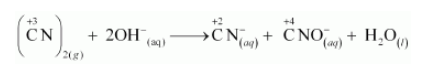# What sorts of informations can you draw from the following reaction ?

Question:

What sorts of informations can you draw from the following reaction ?

$(\mathrm{CN})_{2(g)}+2 \mathrm{OH}_{(2 q)}^{-} \longrightarrow \mathrm{CN}_{((q)}^{-}+\mathrm{CNO}_{(a q)}^{-}+\mathrm{H}_{2} \mathrm{O}_{(l)}$

Solution:

The oxidation numbers of carbon in $(\mathrm{CN})^{2}, \mathrm{CN}^{-}$and $\mathrm{CNO}^{-}$are $+3,+2$ and $+4$ respectively. These are obtained as shown below:

Let the oxidation number of C be x.

$(\mathrm{CN})_{2}$

$2(x-3)=0$

$\therefore x=3$

$\mathrm{CN}^{-}$

$x-3=-1$

$\therefore x=2$

$\mathrm{CNO}^{-}$

$x-3-2=-1$

$\therefore x=4$

The oxidation number of carbon in the various species is:It can be easily observed that the same compound is being reduced and oxidised simultaneously in the given equation. Reactions in which the same compound is reduced and oxidised is known as disproportionation reactions. Thus, it can be said that the alkaline decomposition of cyanogen is an example of disproportionation reaction.